Matlab II

Mary PW Chin 钱碧慧博士
PhD (Wales), MSc (Surrey)
E-mail:

Getting started

Dice

Circles

Histograms

Matlab #2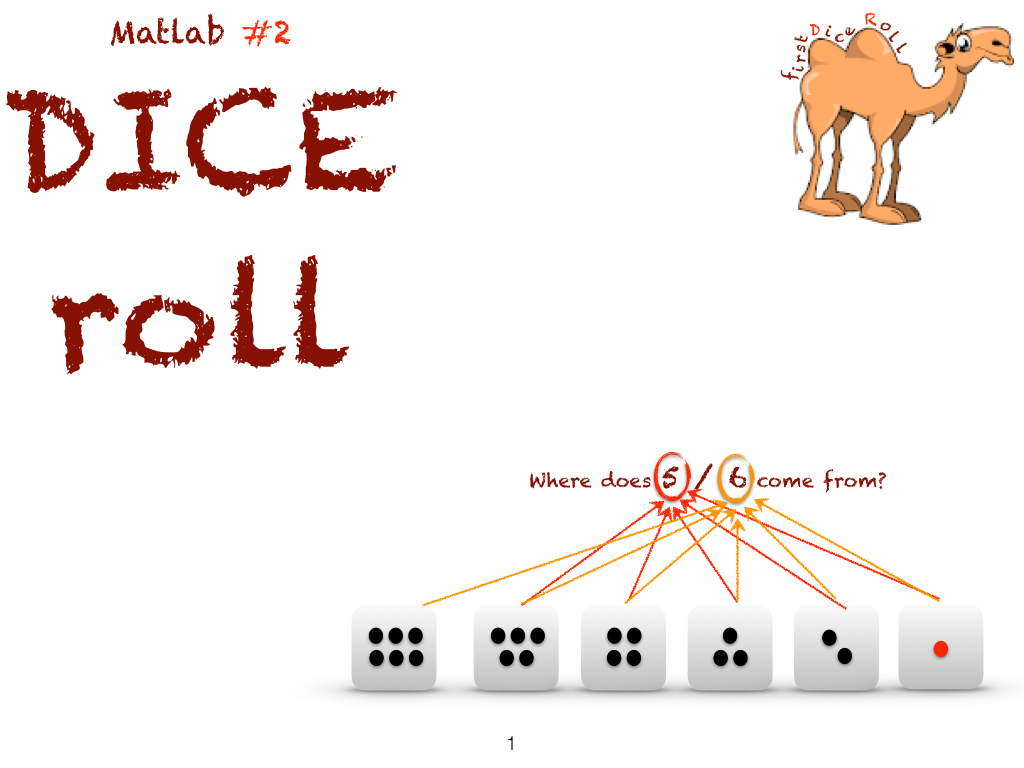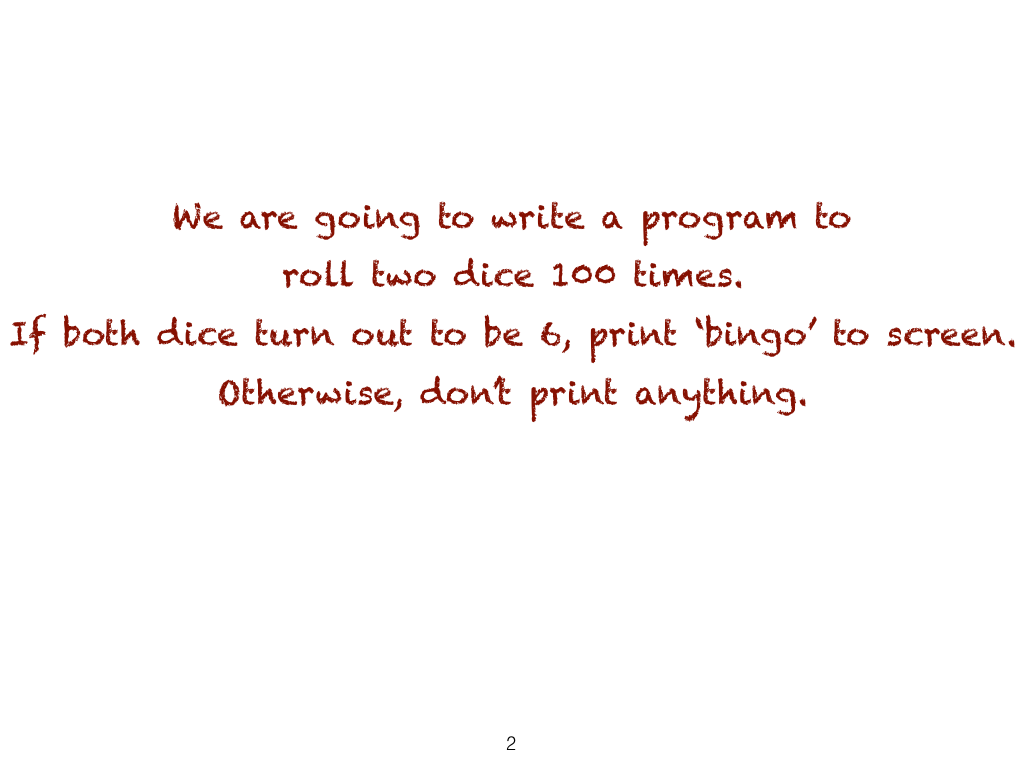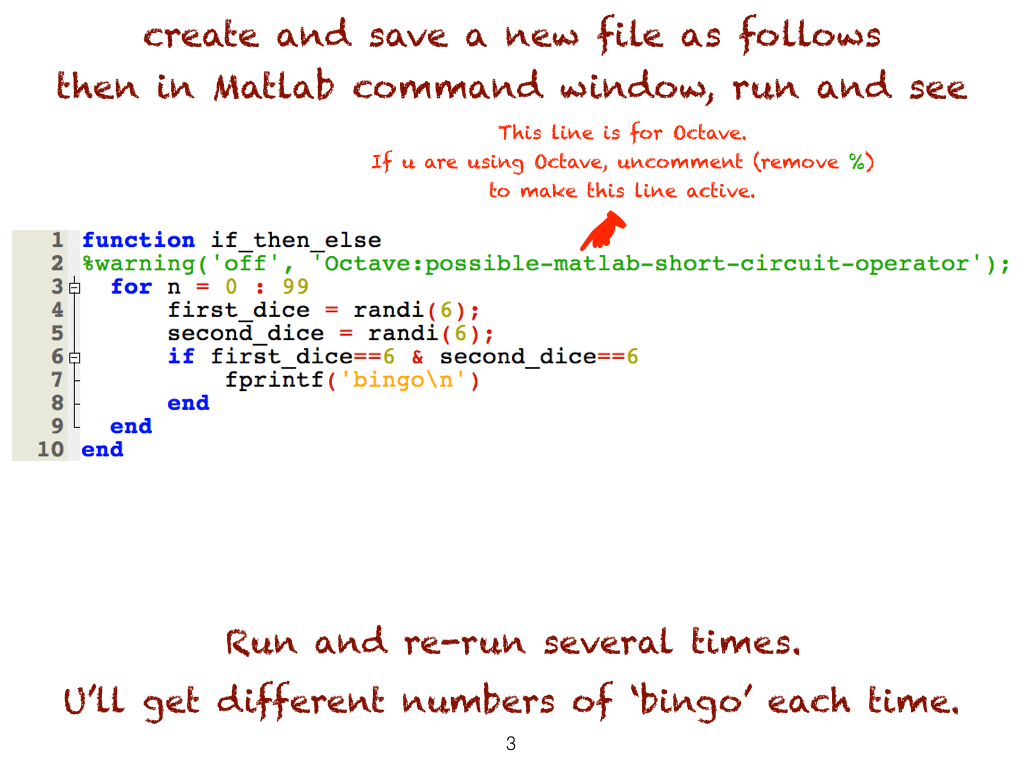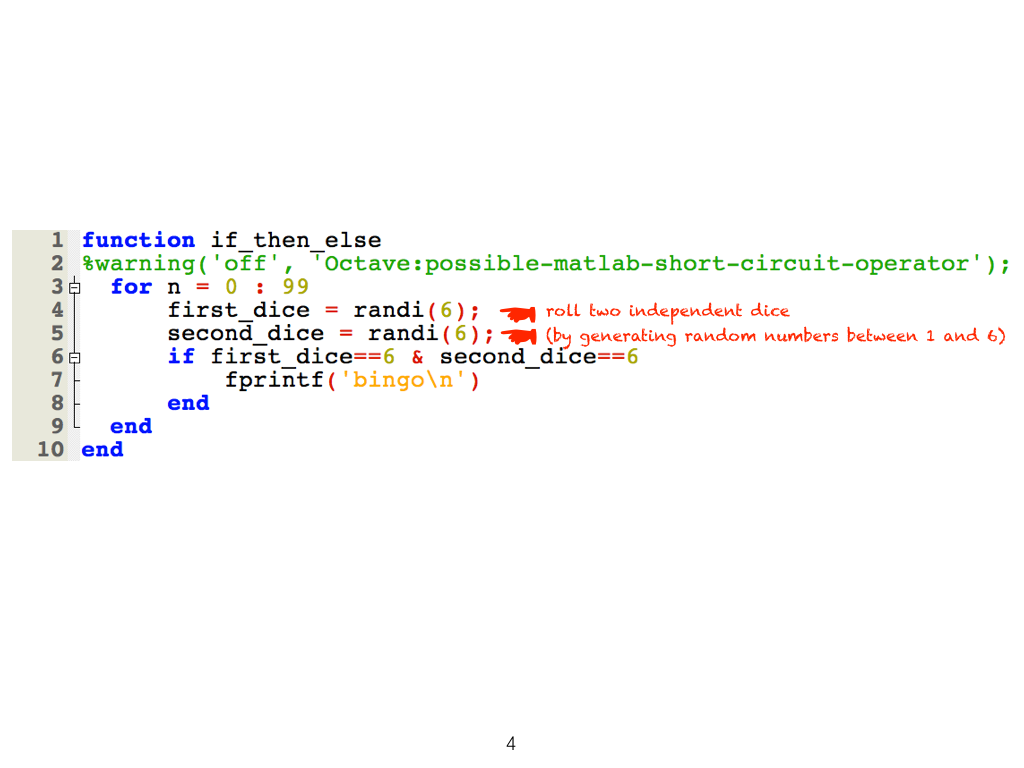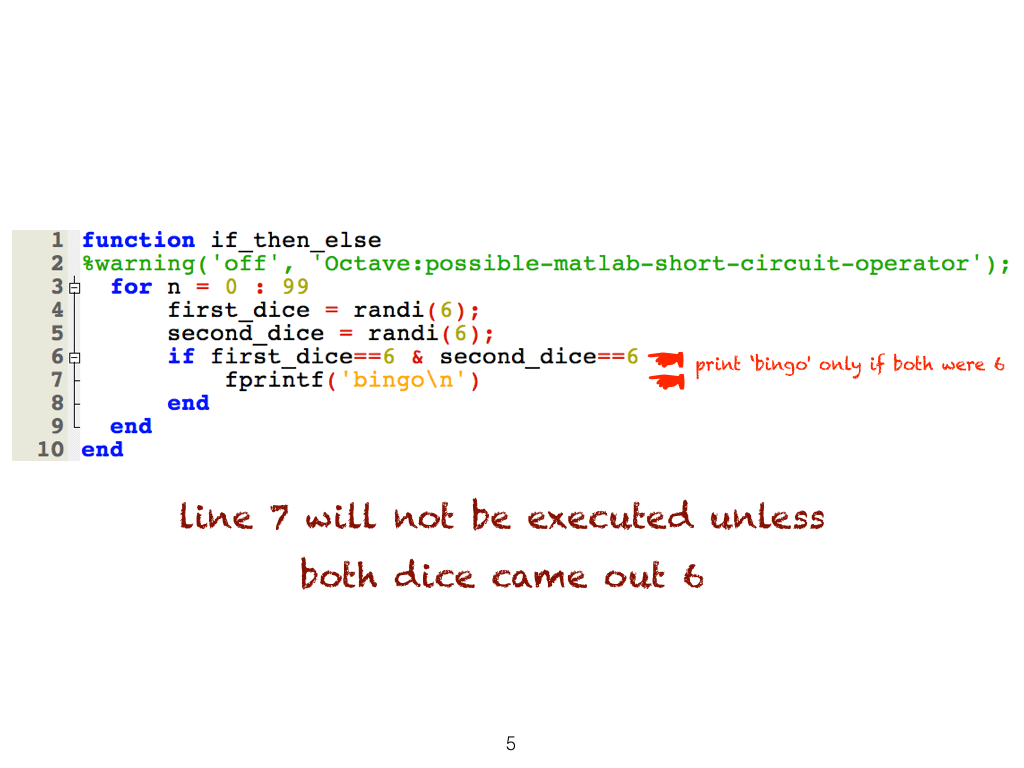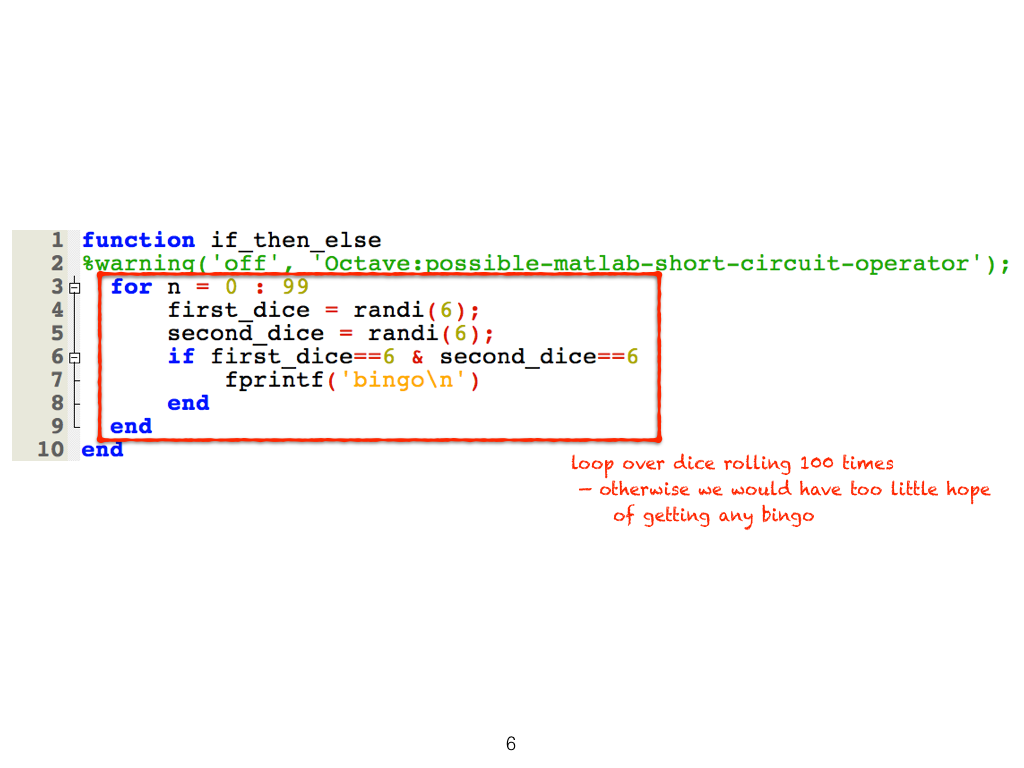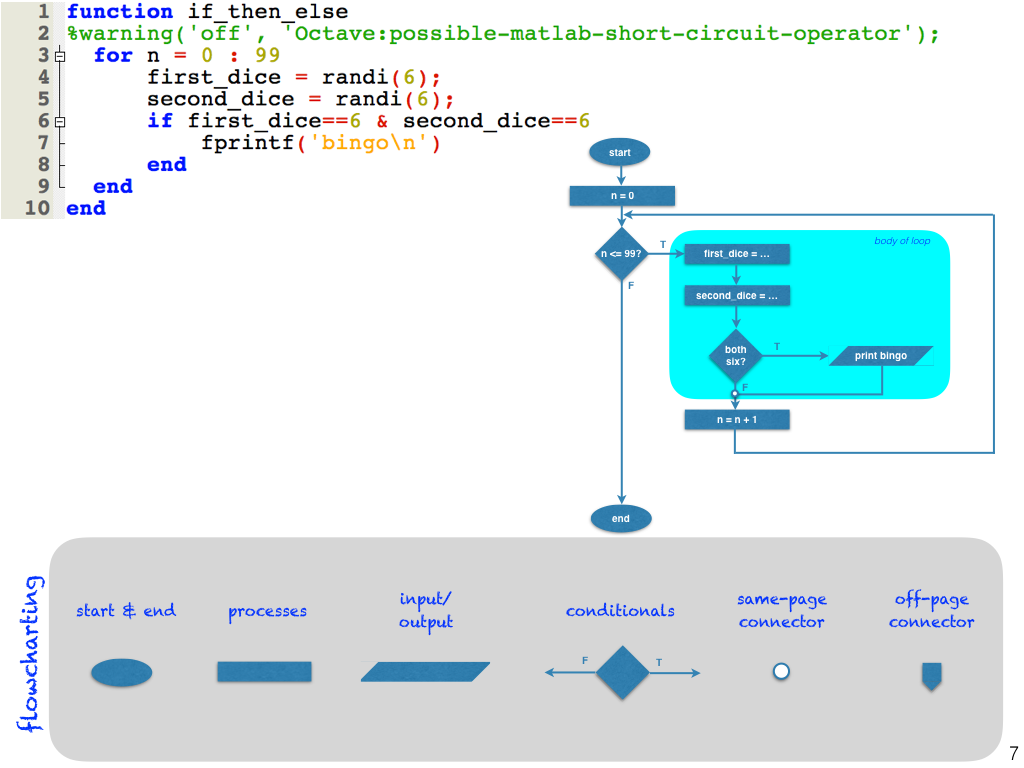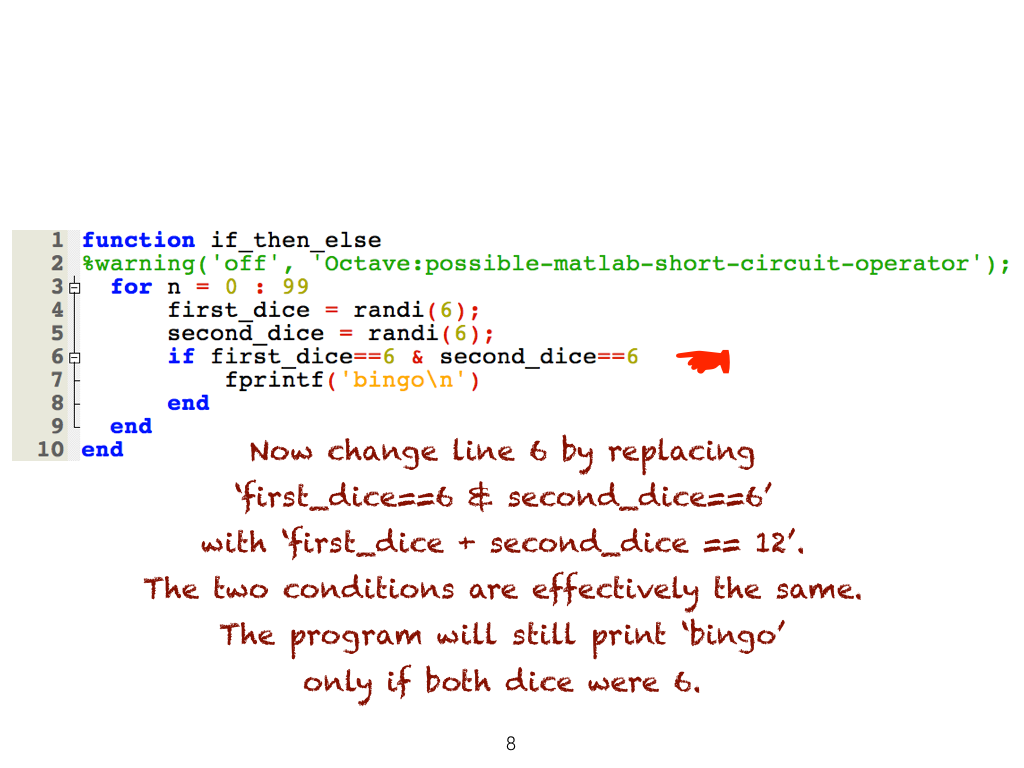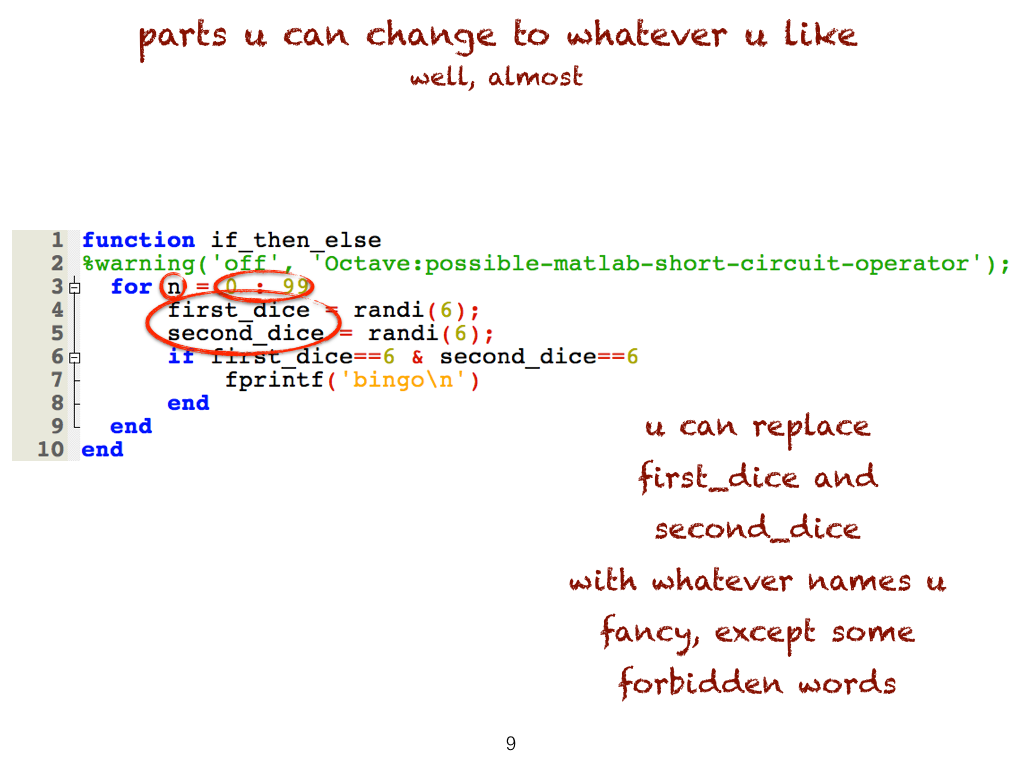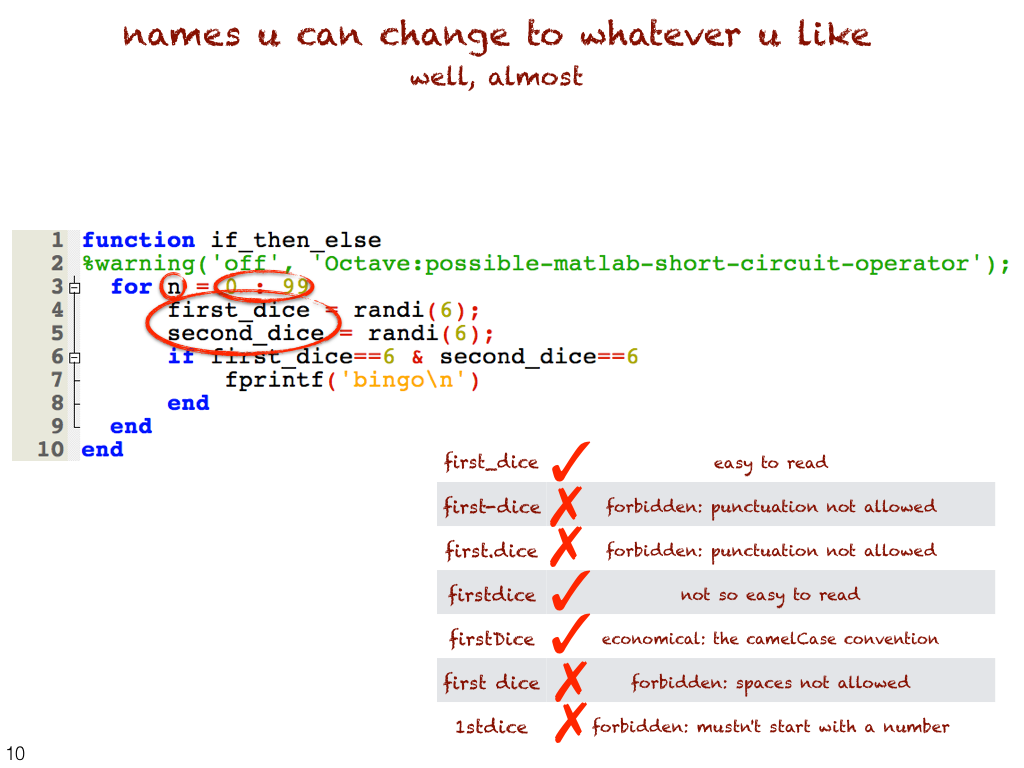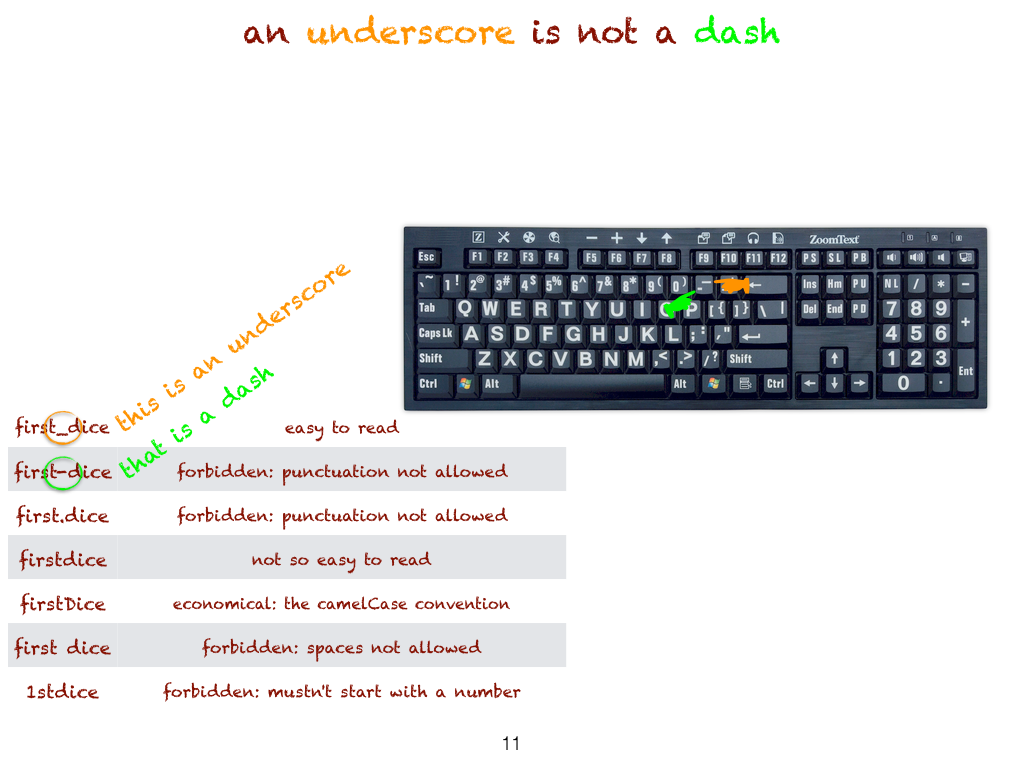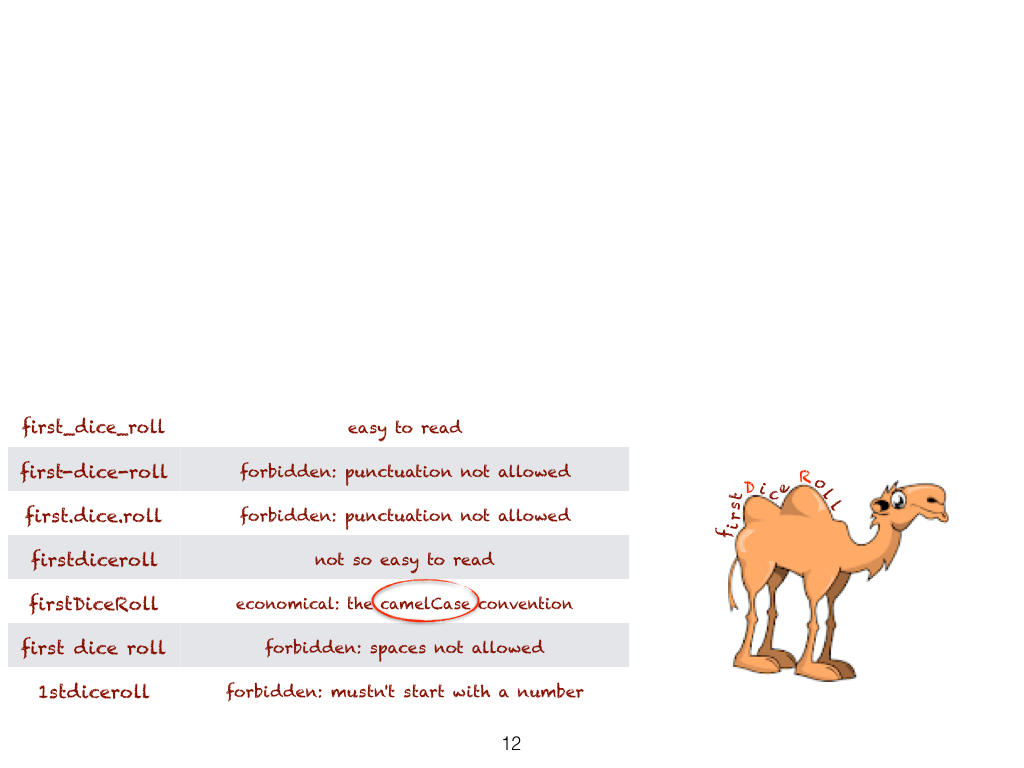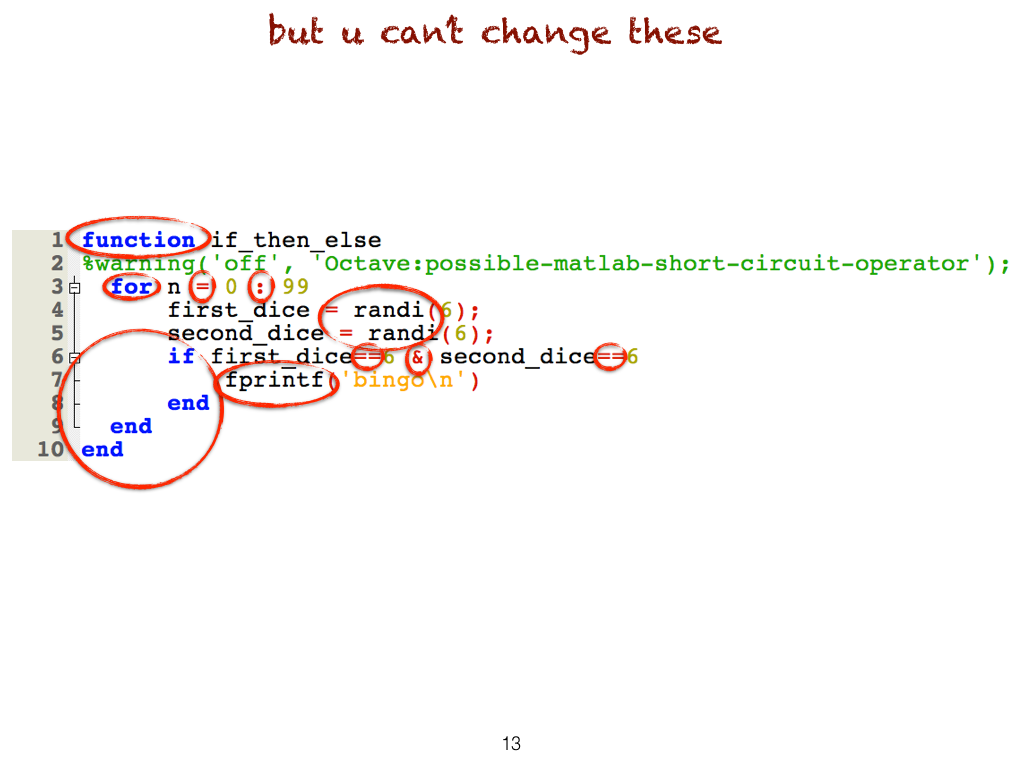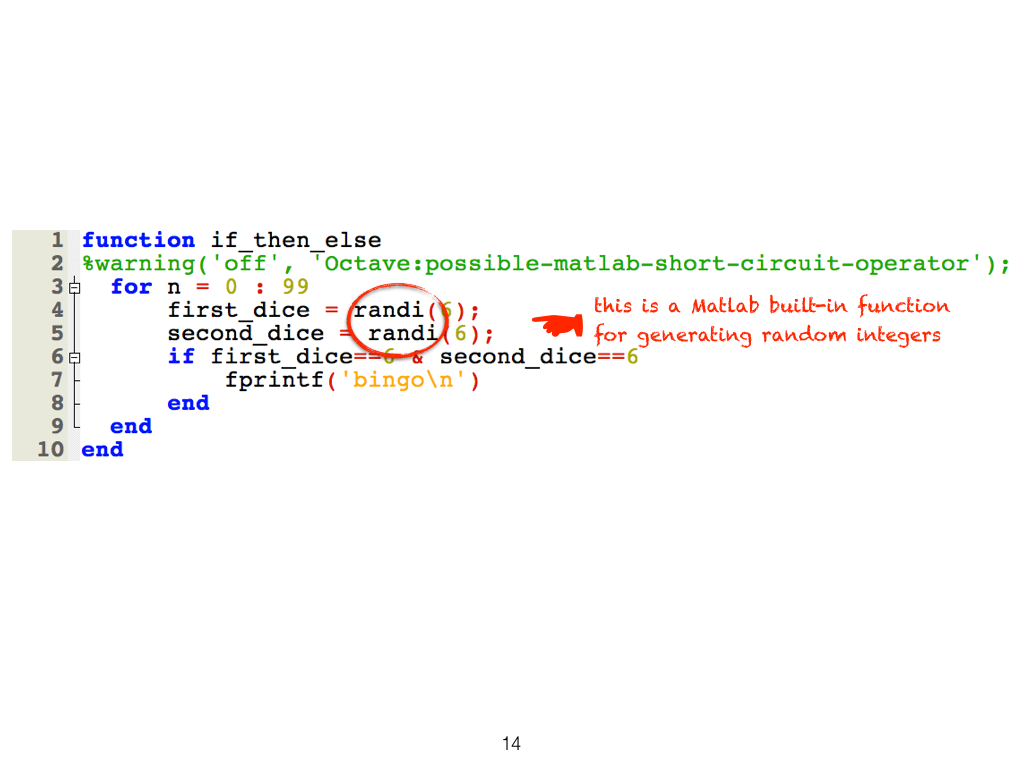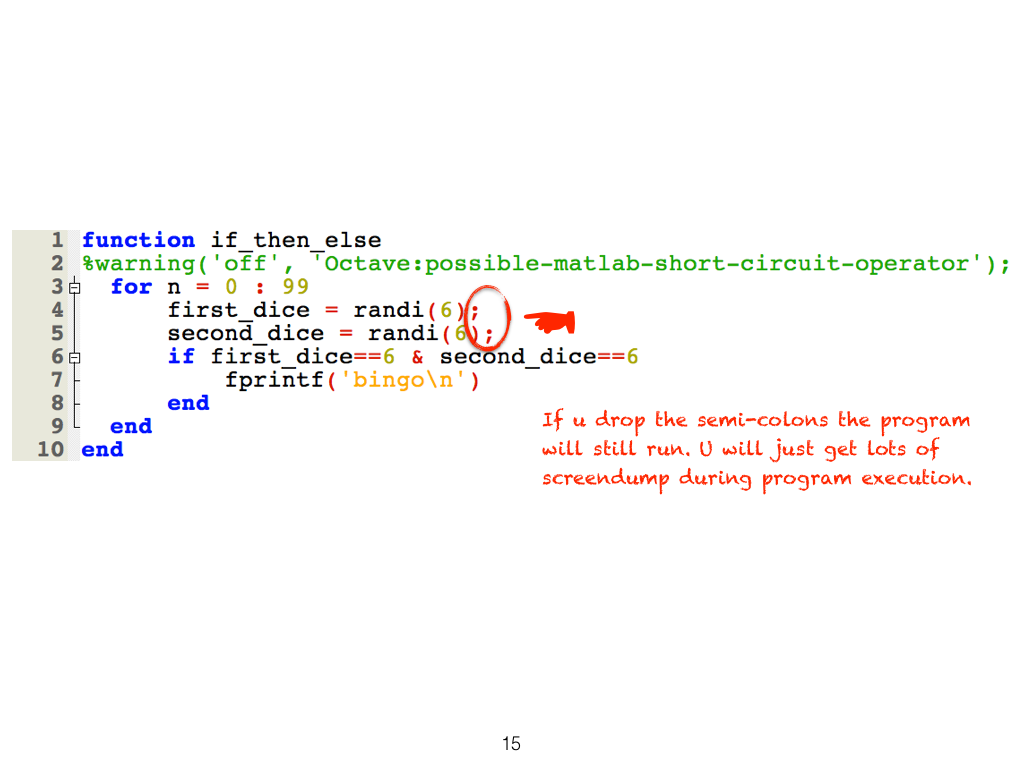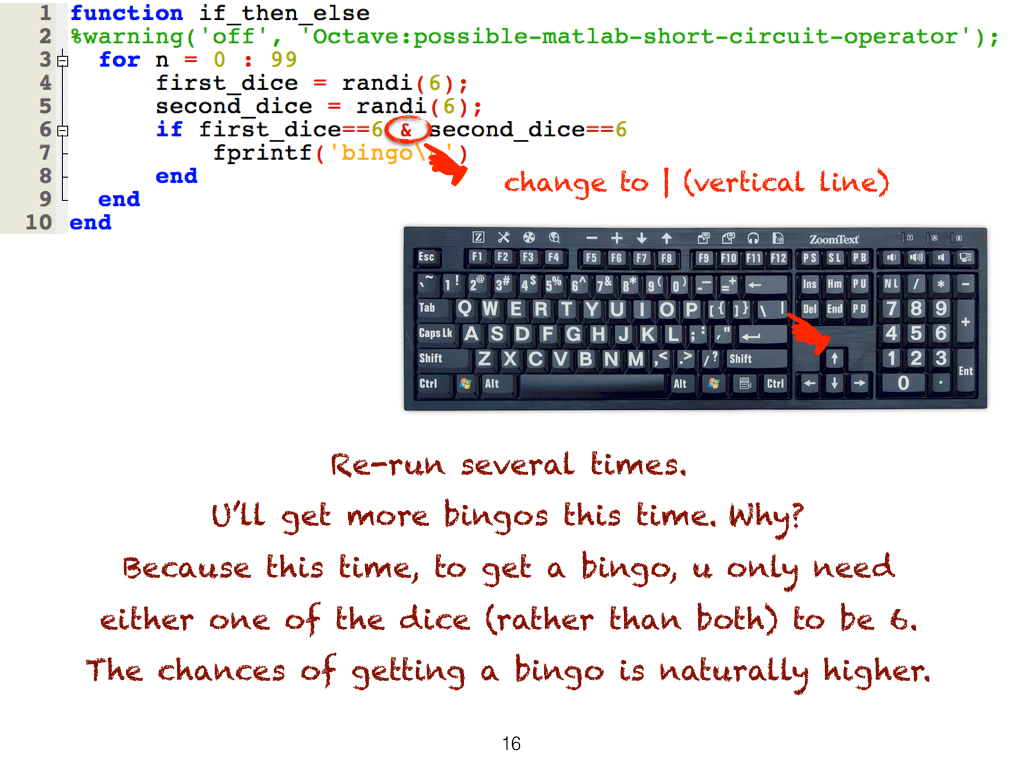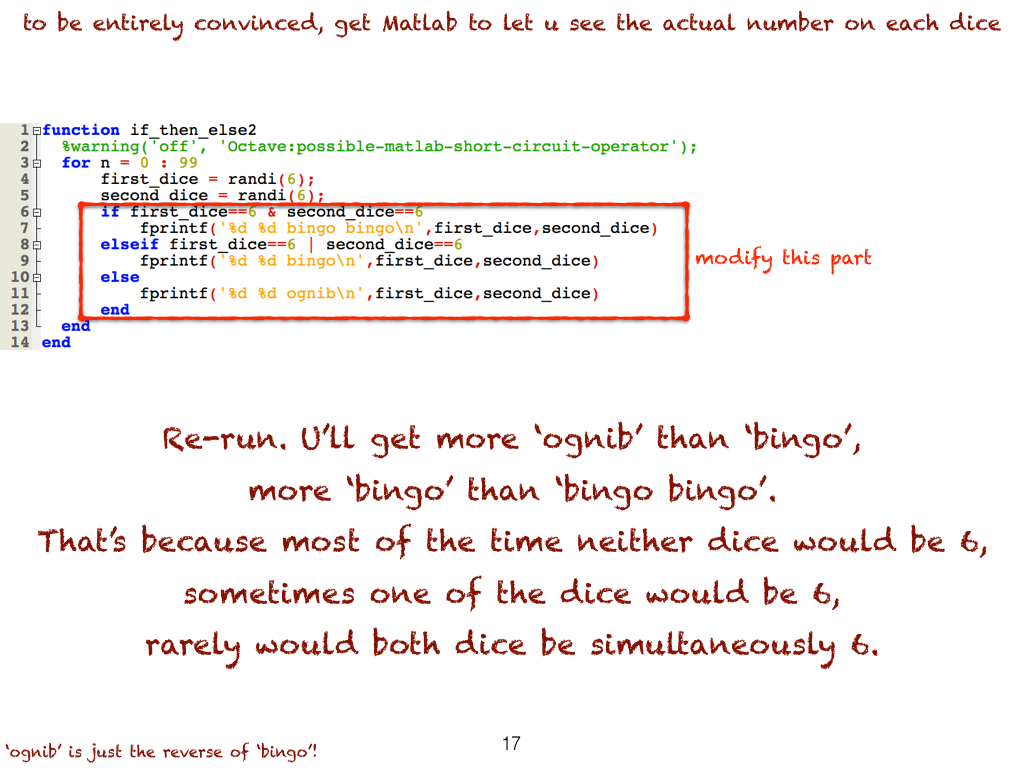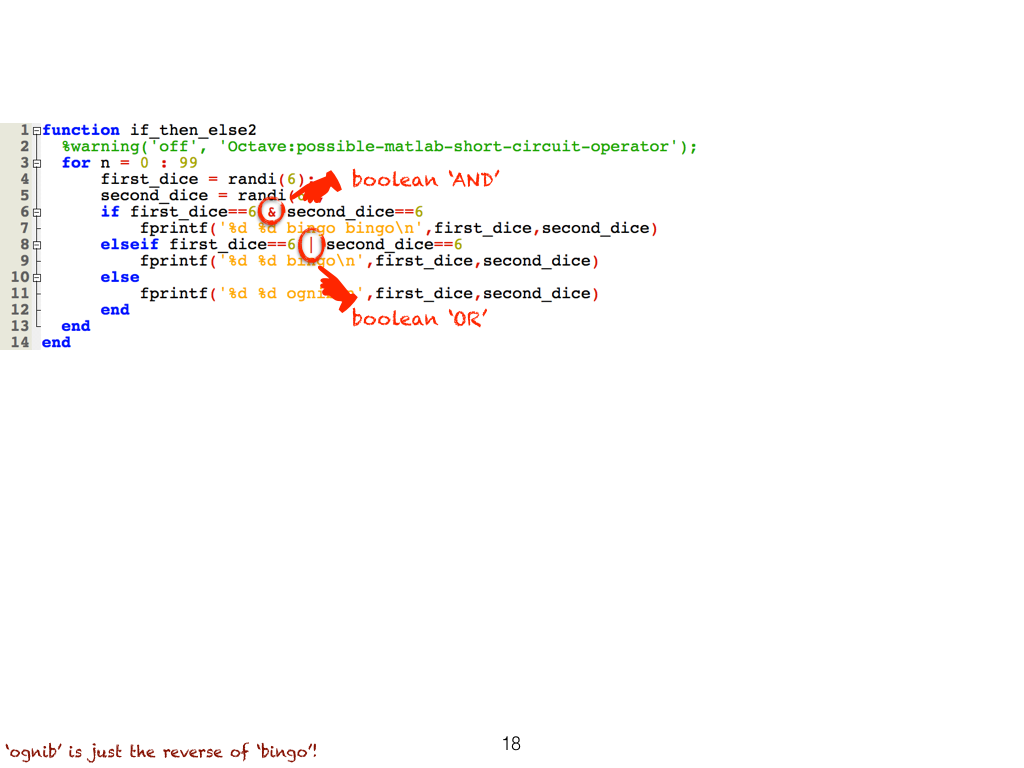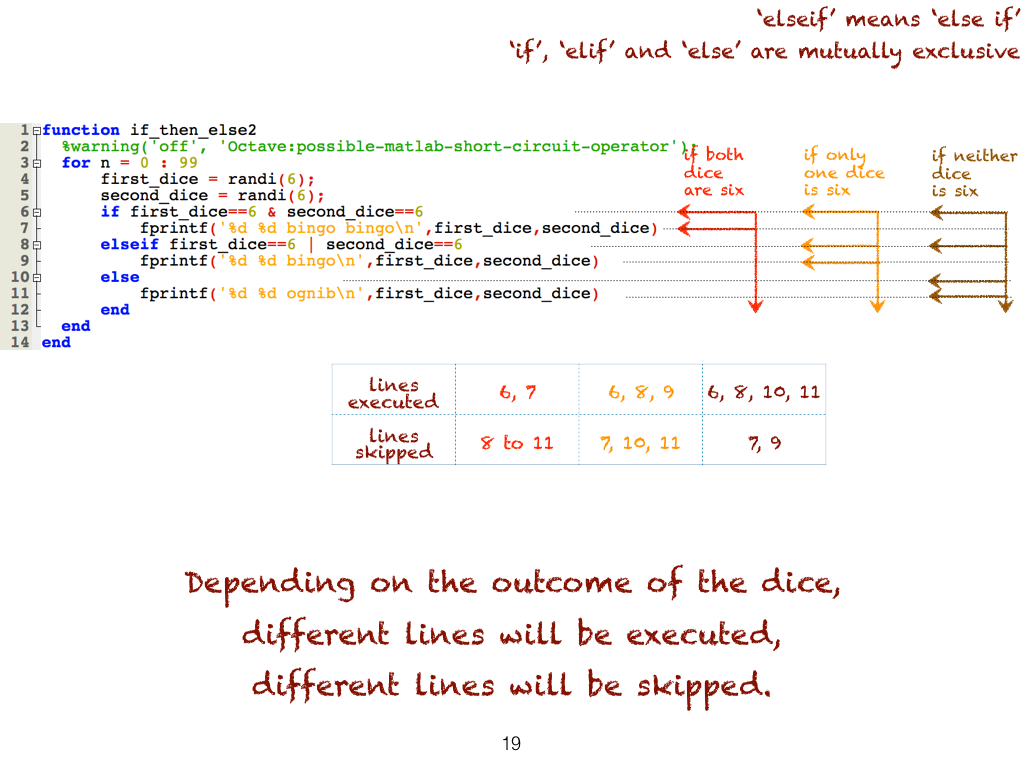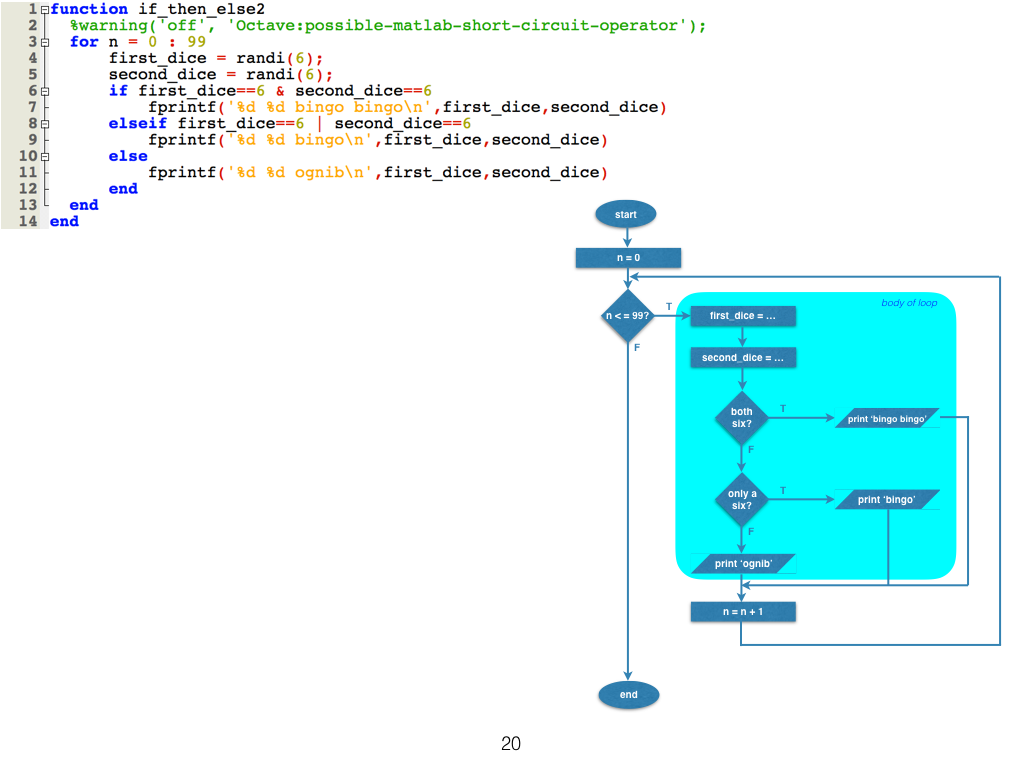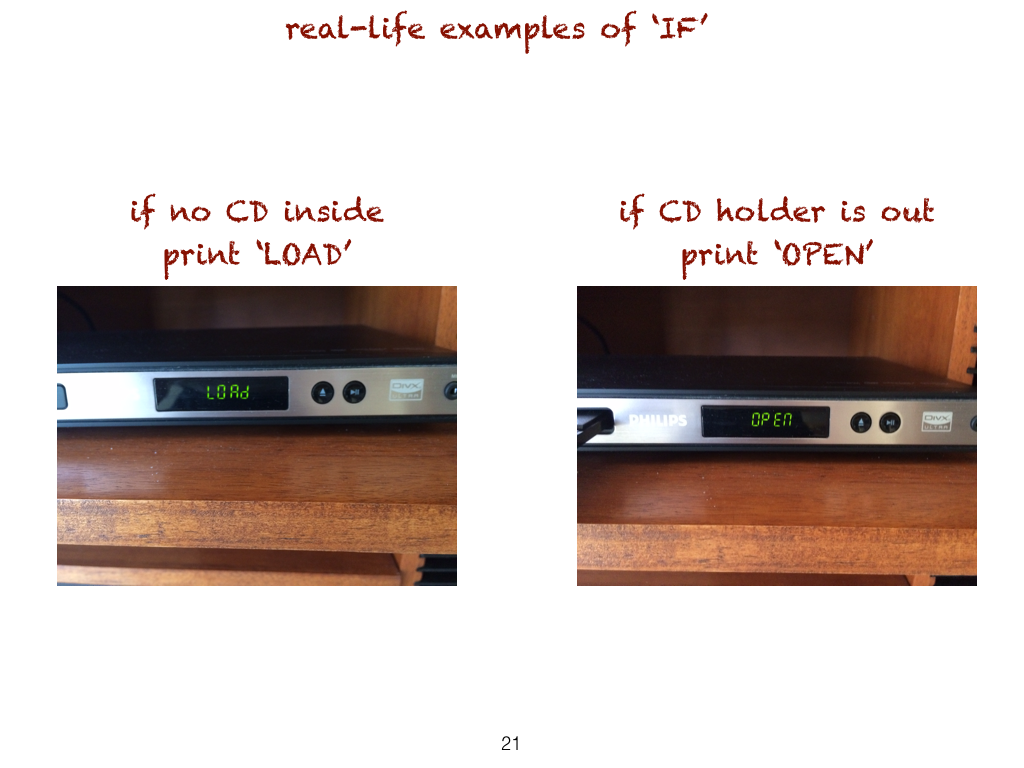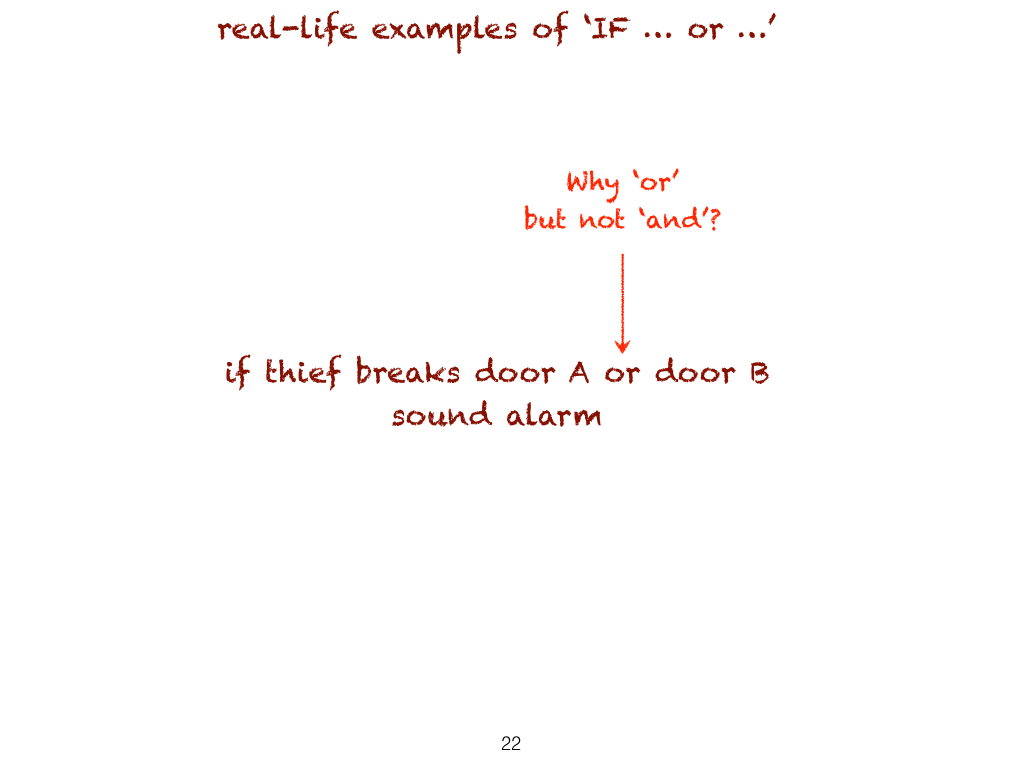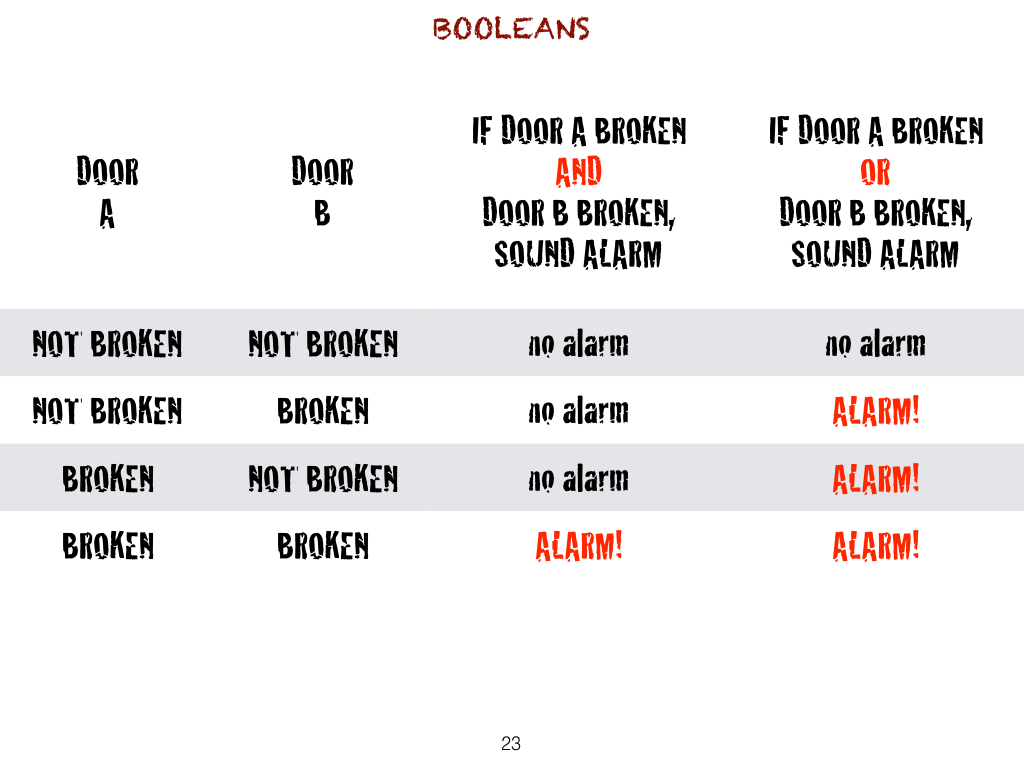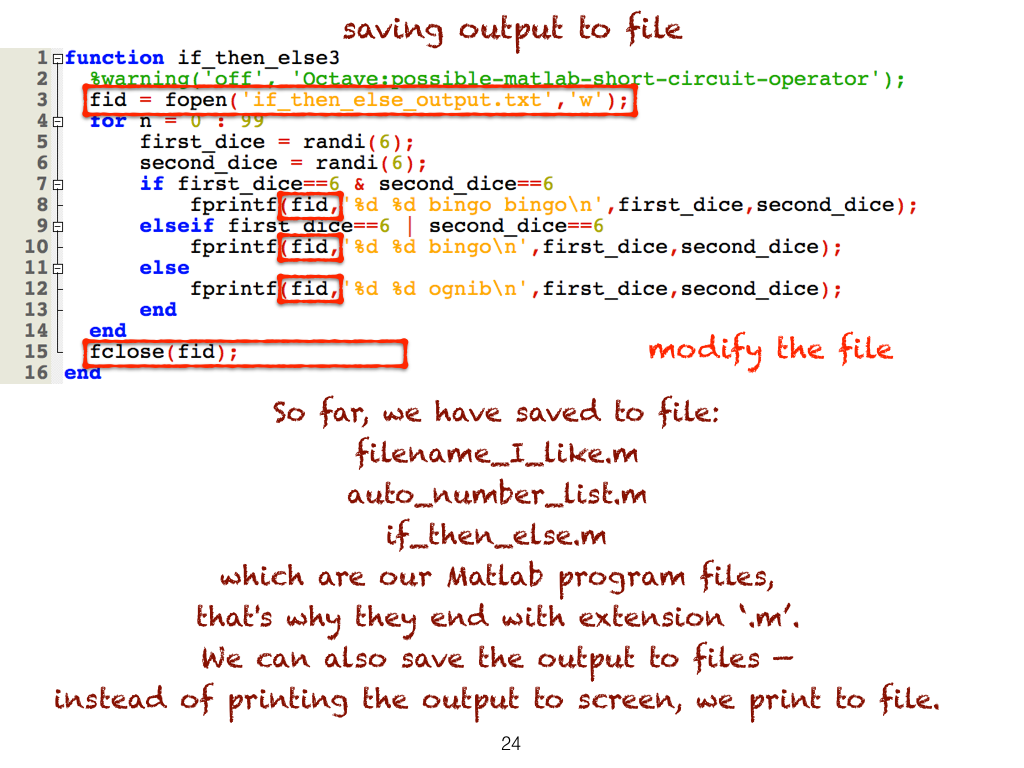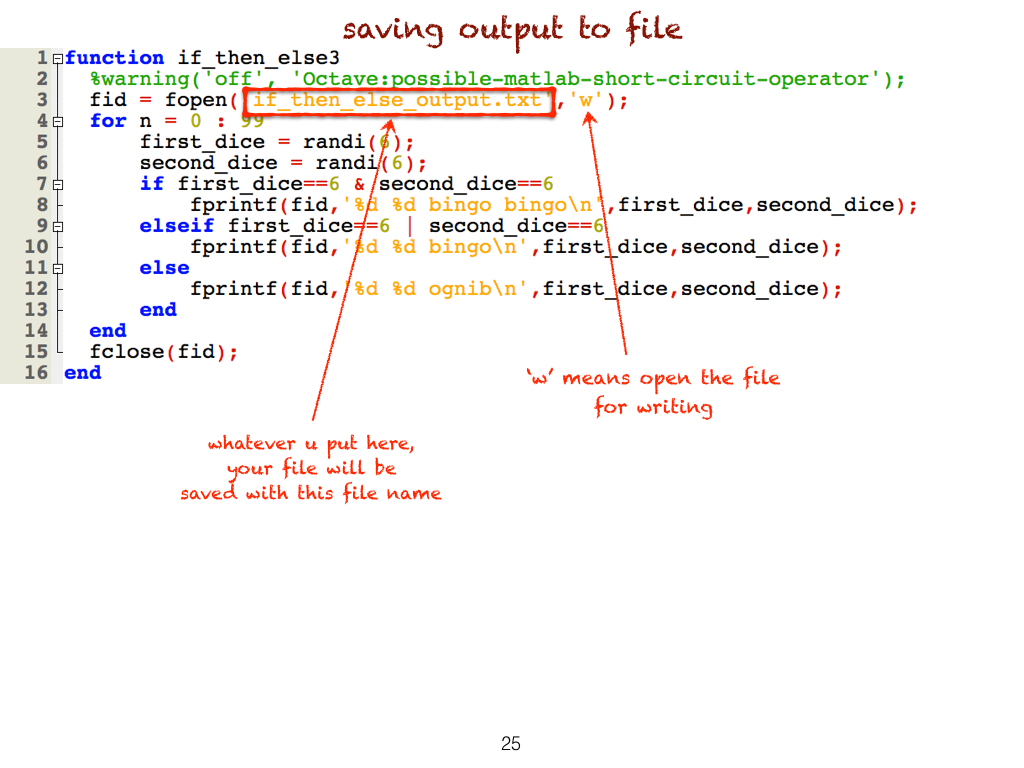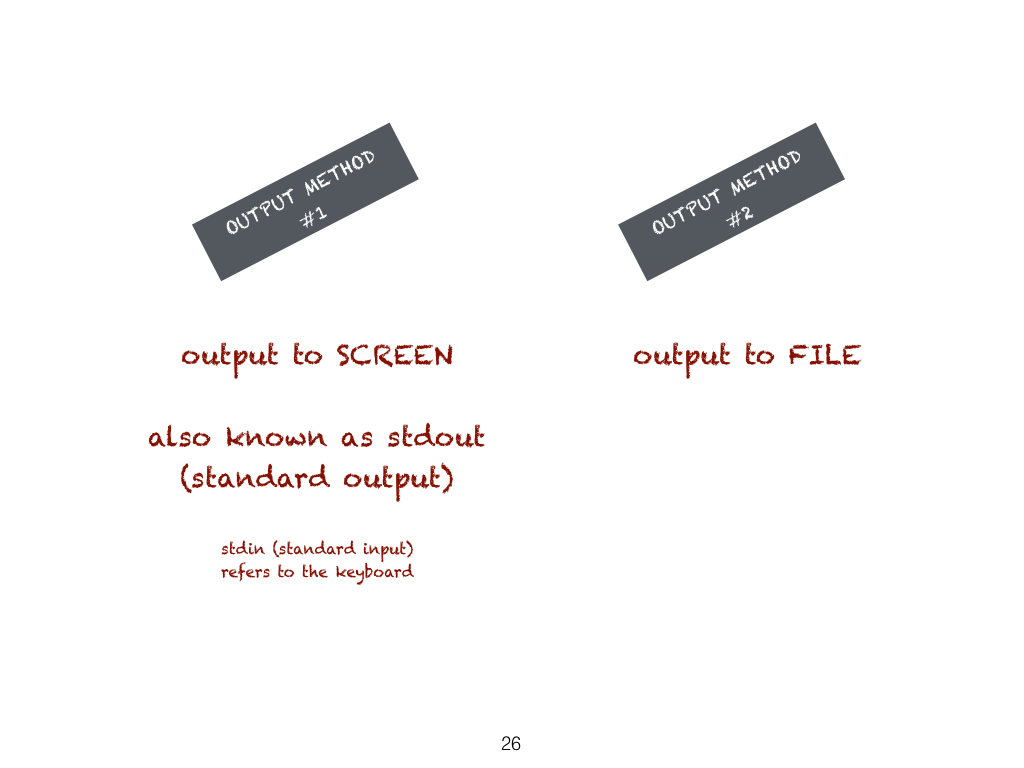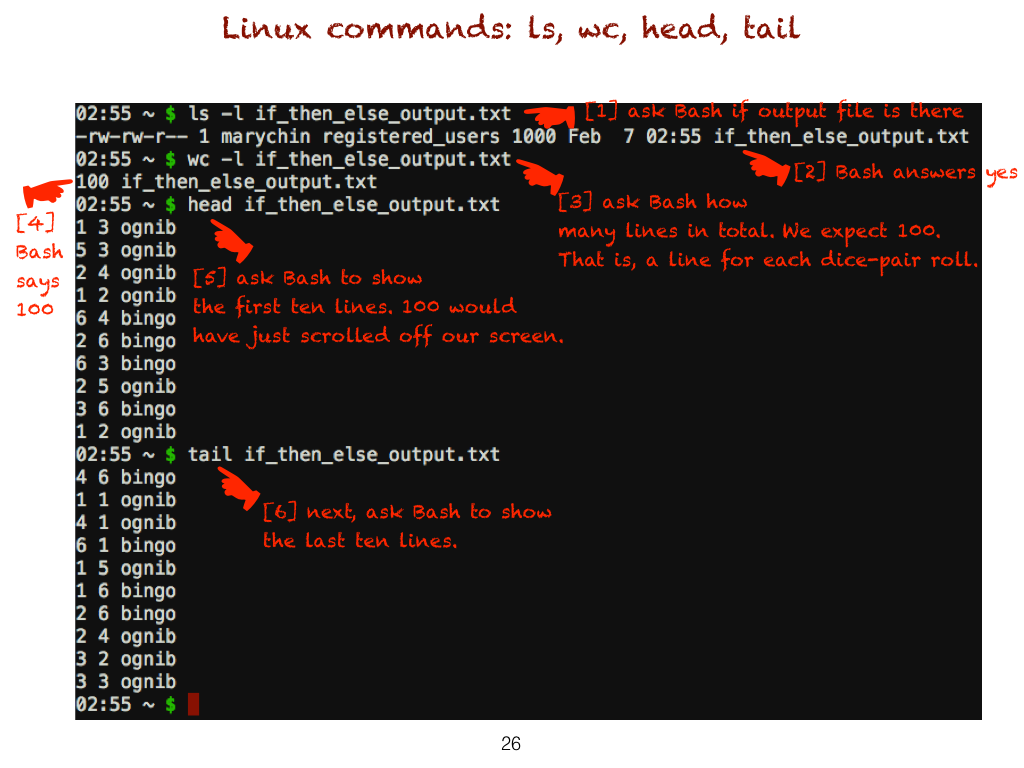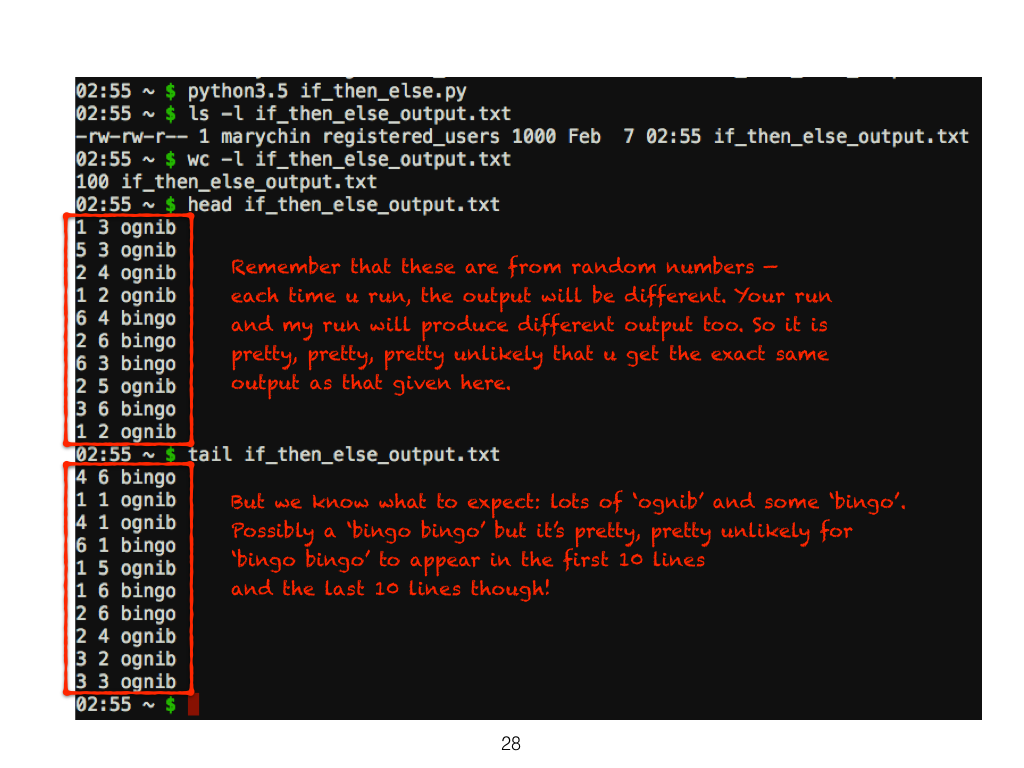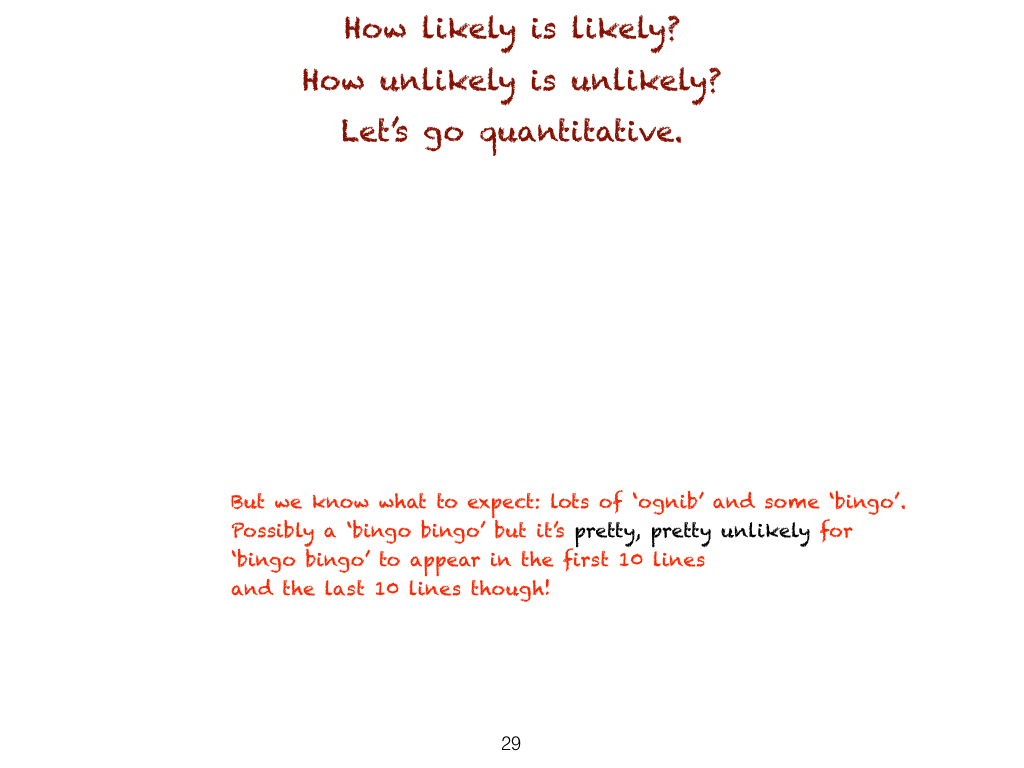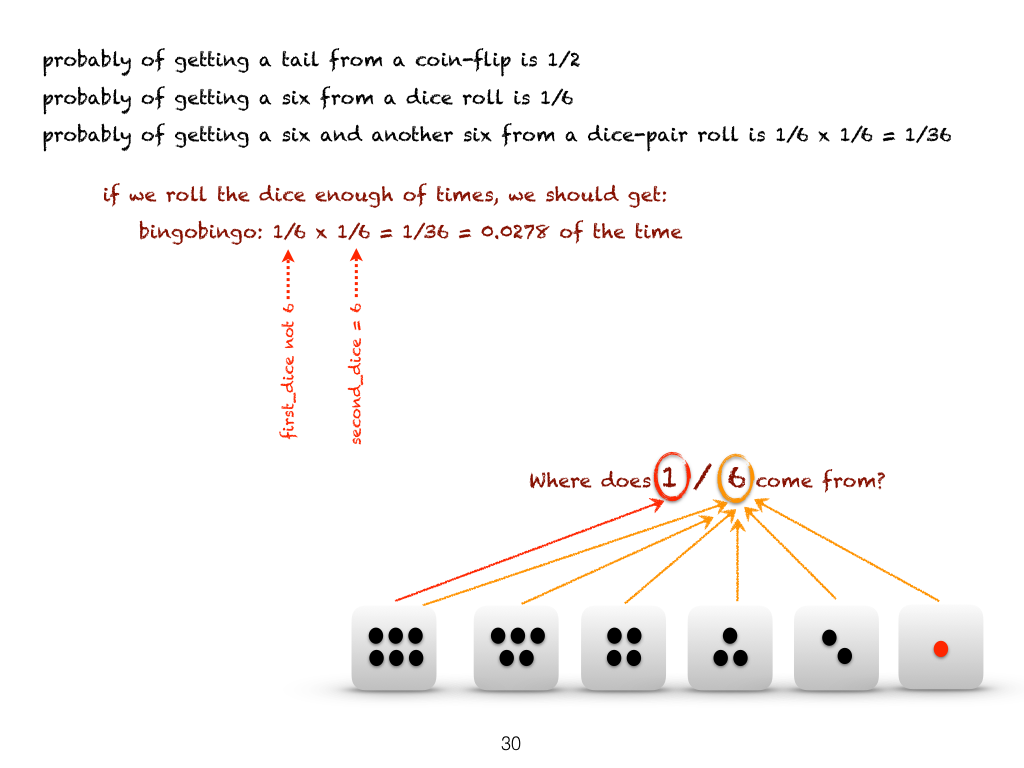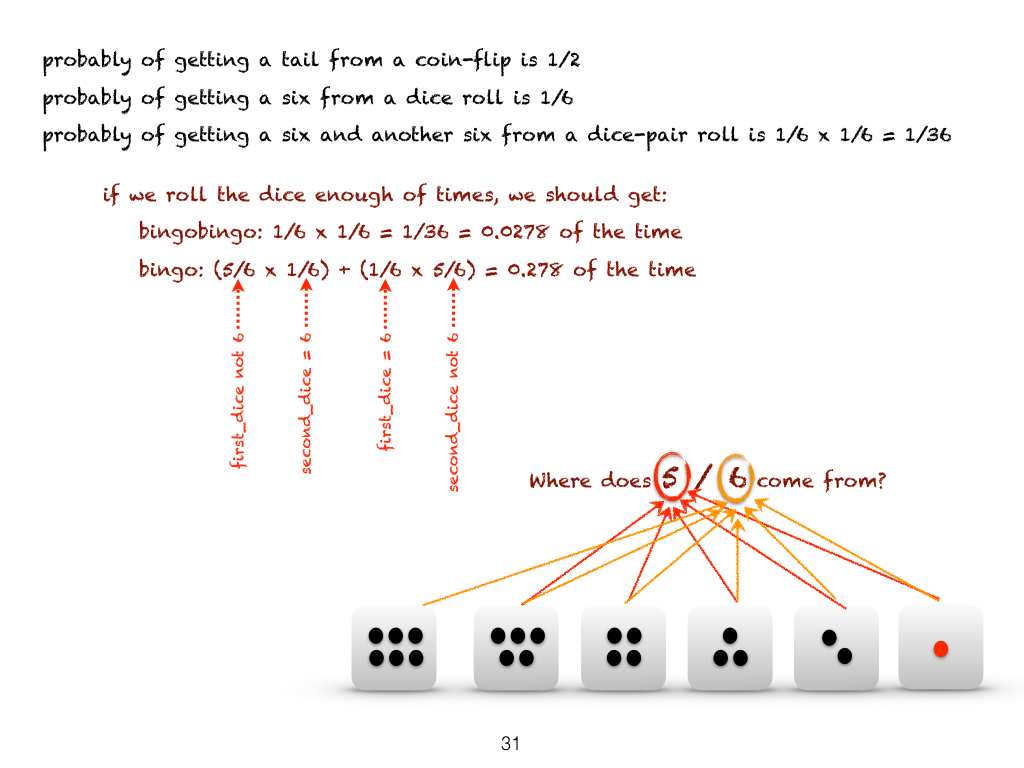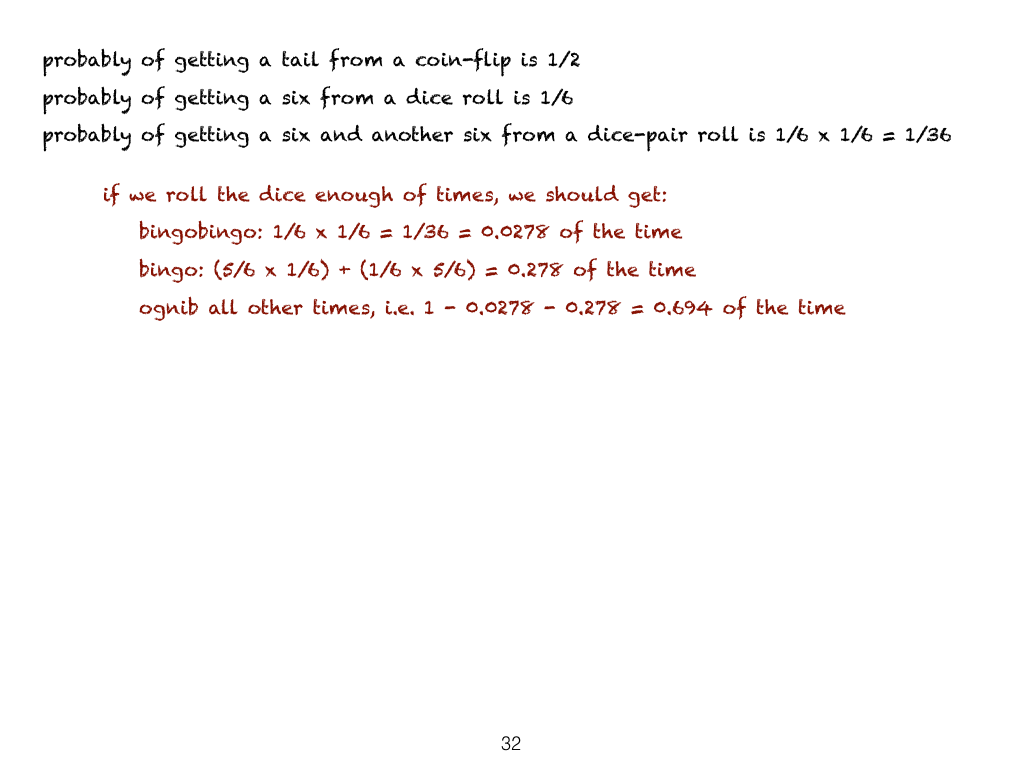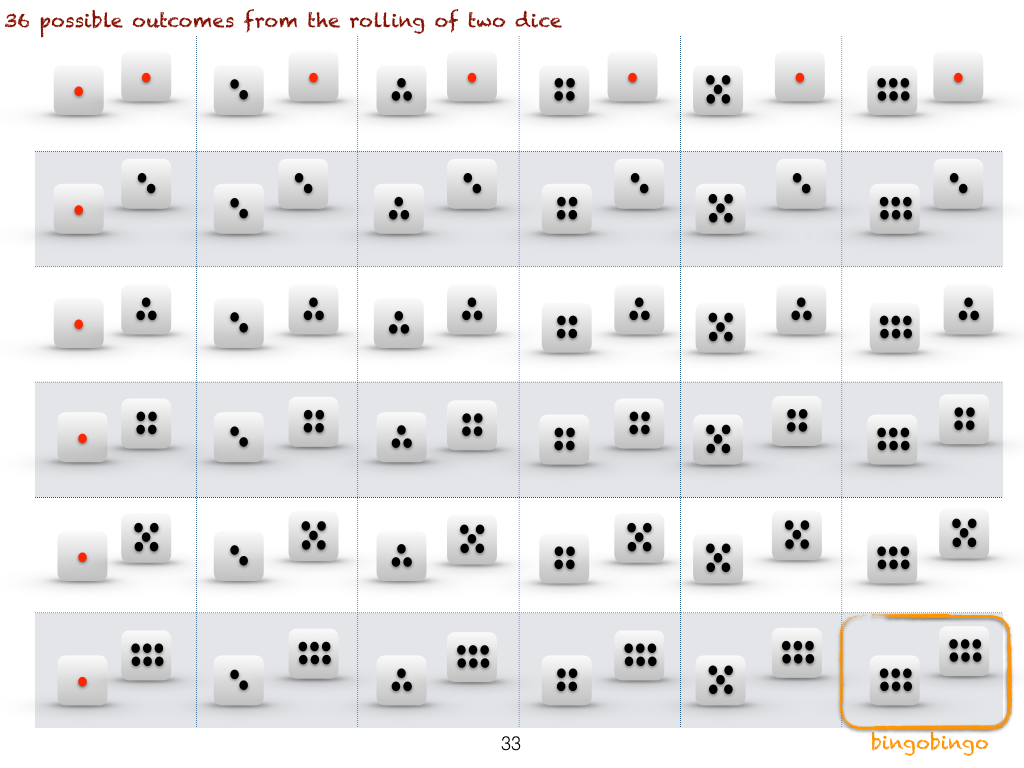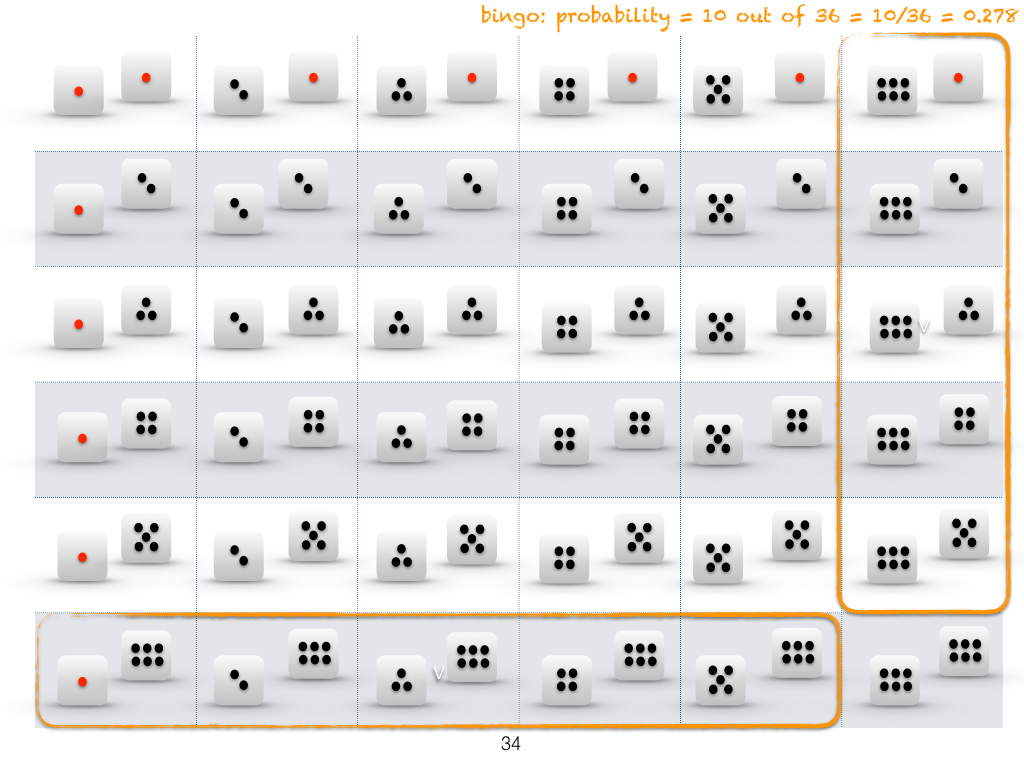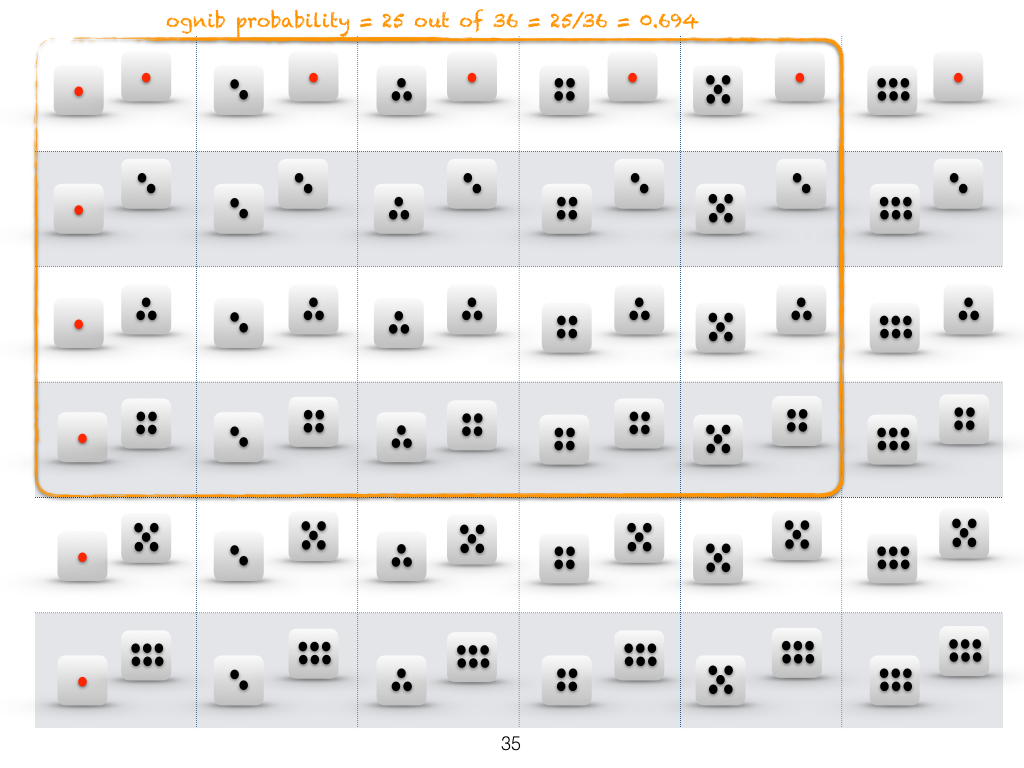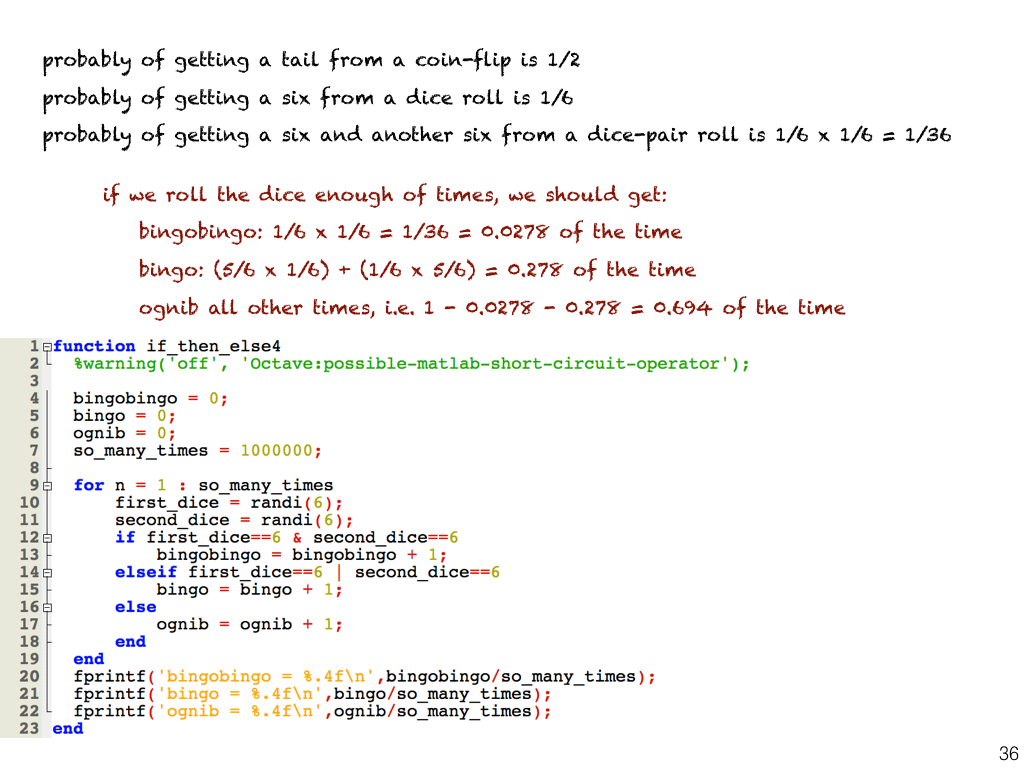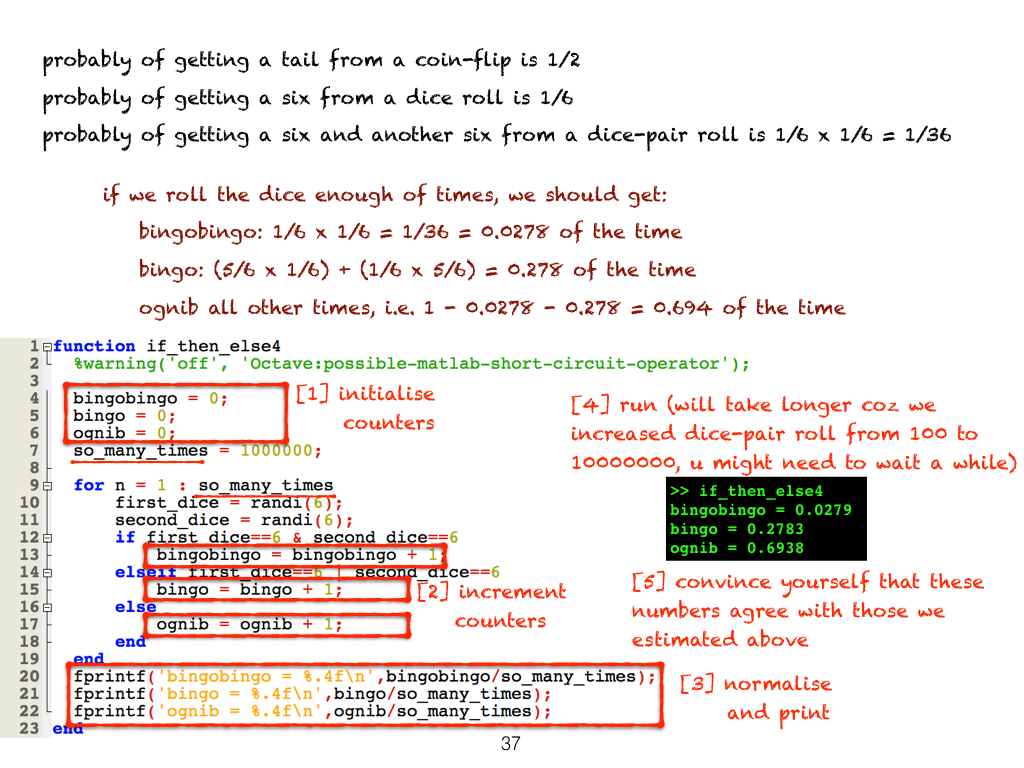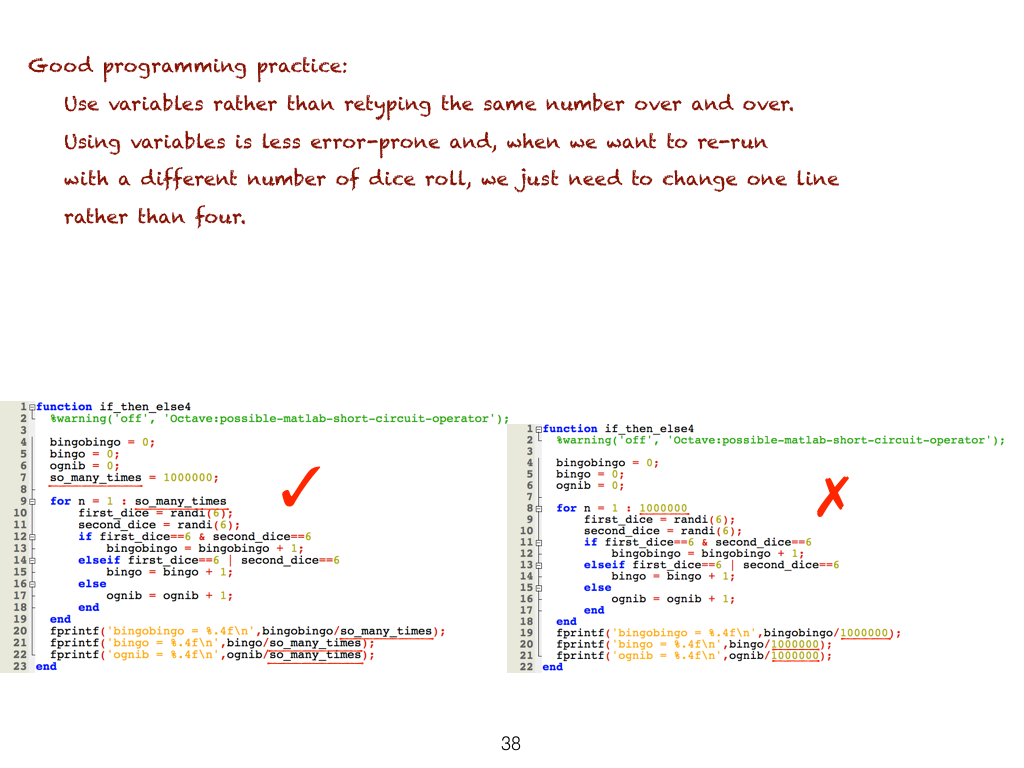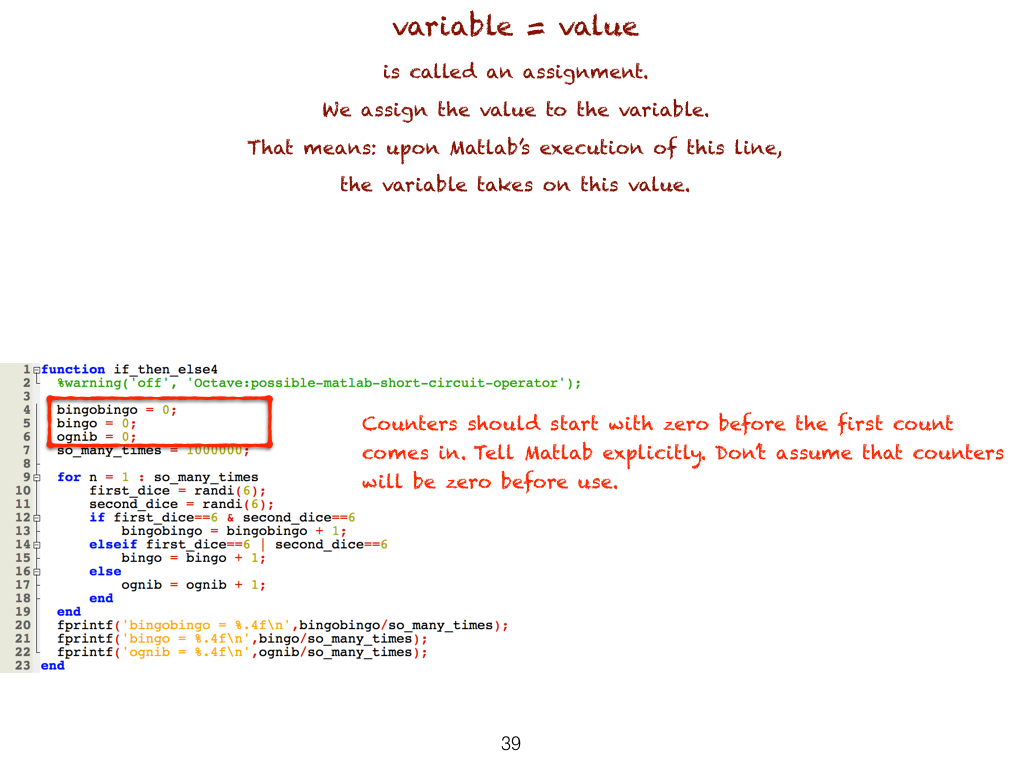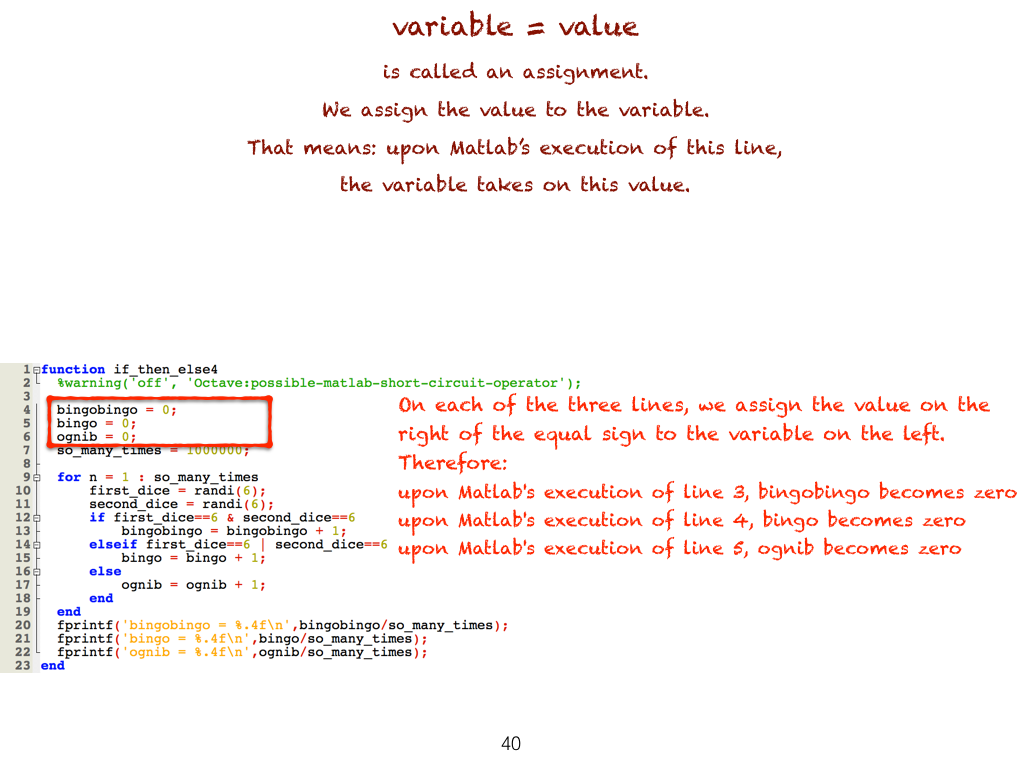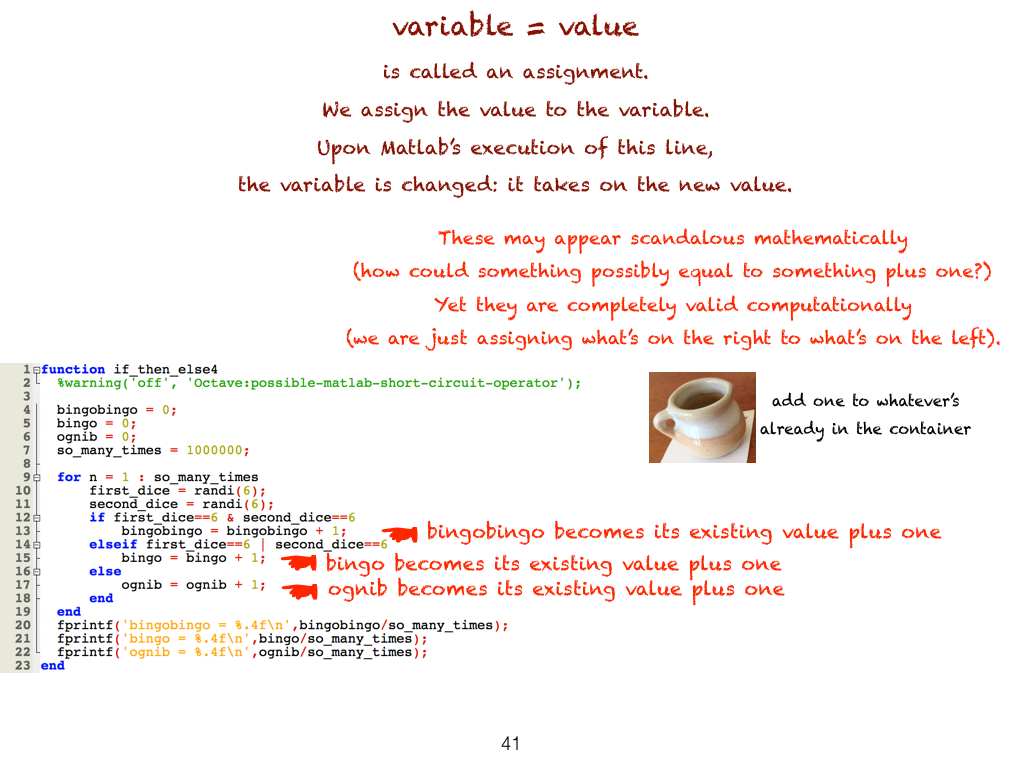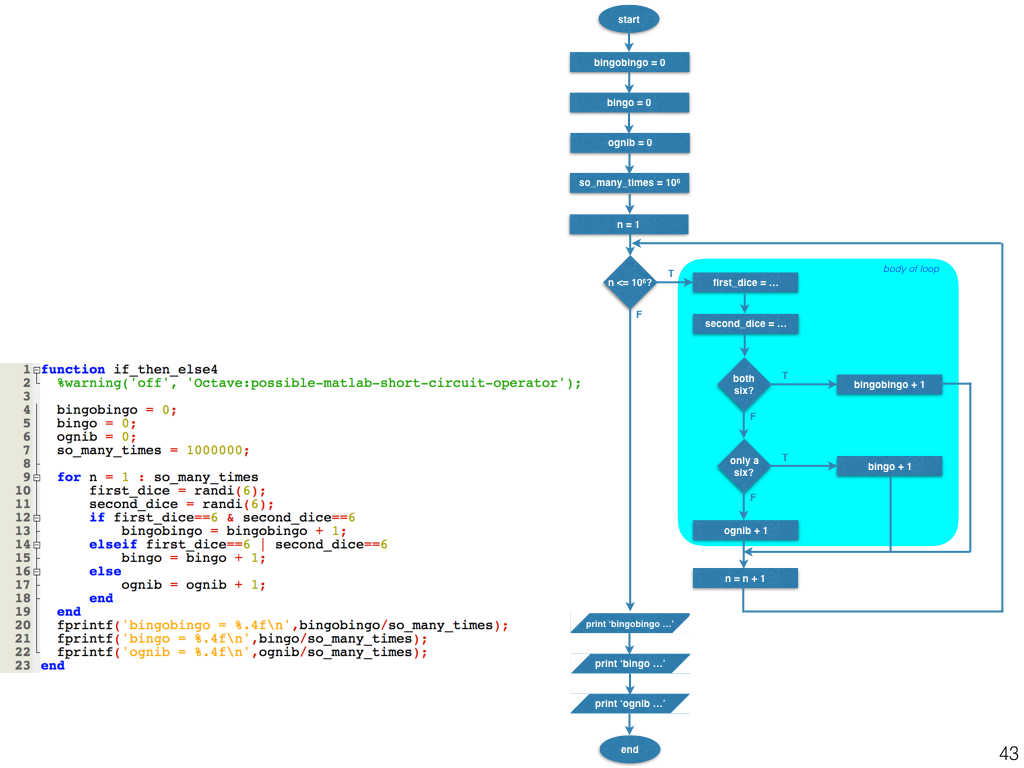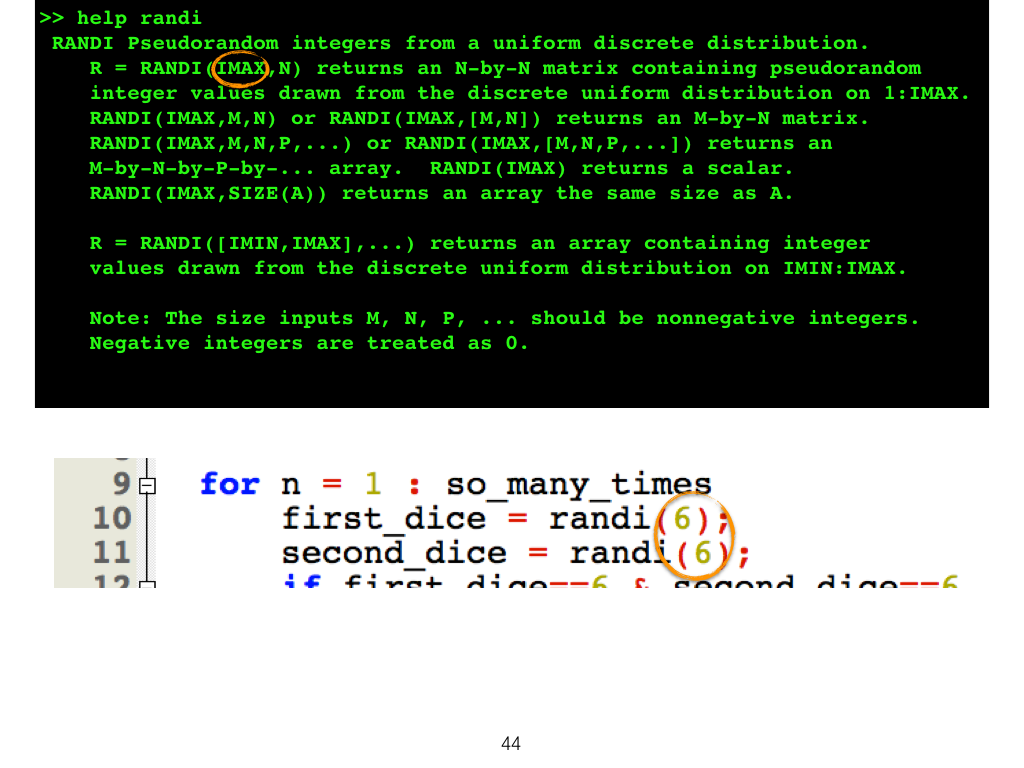Synopsis
1. random numbers and the randi function;
2. conditionals: if-elseif-else;
3. assignment vs comparison;
4. booleans and vs or;
5. variable names: rules and conventions;
6. output to screen (standard output) vs output to file;
7. Linux commands: ls, wc, head, tail;
8. probability theory;
9. initialisation and counters.

Exercise
1. For the program on slide #20 mark out the:
• variable names;
• arguments;
• functions;
• keywords;
• modules;
• methods;
2. Repeat Exercise 1 for the program on slide #24.
3. Repeat Exercise 1 for the program on slide #36.
4. Refer to the program on slide #36. What difference would you expect if we change
for n = 1 : so_many_times to
• for n = -99 : so_many_times-100;
• for n = so_many_times+1000 : -1 : 1001;
• for n = 1 : 1000 : so_many_times*1000?
5. Refer the program on slide #40. What happens if we change elseif to if? Draw the revised flowchart.
6. Write a program to generate two random numbers between 0 and 100. Print the larger number, and the sum, difference and product of the two numbers.
7. Write a program to generate a random number between 0 and 10. Take the number as the radius of a circle. Print the diameter, circumference and area of this circle.
8. Write a program to generate a random number 1930 ≤ y ≤ 2017, take this as somebody's year of birth. Generate another random number 1 ≤ m ≤ 12, let this be his month of birth. We need to know his age in the coming years until he reaches 90. Print your output in three columns:
YEARMONTHAGE
20181__ years __ months
20182
20183
____90 years 0 month

9. Write a program simulating the draw of Magnum 4D first prize.
10. Write a program simulating your favourite chance-based game.

Getting started

Dice

Circles

Histograms